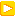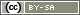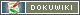# Introduction to Arrays

Arrays are an extremely useful tool in PHP. They are predominately used when filters, rotation, or analysis cannot be carried out by using standard functions. You can find concrete examples of the PHP code used in their respective chapters, e.g. Rotation, Count Points for Answers, Use Selected Items In Another Question.

## Arrays

An array consists of a list of values (e.g. numbers or text). The following PHP code generates a simple array with three list items (in this example; text): “one”, “two”, and “three”. The array is subsequently stored in the `\$a` variable.

```// create an array
\$a = array('one', 'two', 'three');```

Instead of the notation `array()` a short notation with square brackets is also possible. The function is identical.

```// Create an array with square brackets
\$a = ['one', 'two', 'three'];```

The list generated as a result can be seen below. A number is assigned to each item in the list (the so-called index or key), which normally begins with 0. The length of this list is 3.

 Value Index one two three 0 1 2

Individual items in the array can now be accessed using square brackets, (`[]`) . The index is written inbetween the brackets. The command `count()` reveals something about the array length.

```// work with arrays
\$a = array('one', 'two', 'three');
html('<p>Length of array: '.count(\$a).'</p>');
html('<p>Element with index 0: '.\$a.'</p>');
html('<p>Element with index 2: '.\$a.'</p>');```

The example code generates the following output in the questionnaire:

```Length of array: 3
Entry with index 0: one
Entry with index 2: three```

Tip: Normally, you cannot see directly what was saved in an array. Use the `debug()` function in order to view this. If you start the questionnaire in debug mode (yellow arrow), `debug()` then generates a visual output.

```\$a = array();
for (\$i=0; \$i<10; \$i++) {
\$a[] = \$i + 1;
}
debug(\$a);  // only visible in debug mode!```

## Build Arrays Piece by Piece

In PHP, the option to add an element to the array is particularly useful. In order to do so, empty square brackets are written after the array variable, and then, after an equals sign, what should be added to the array:

```// expand arrays
\$a = array('one', 'two', 'three');
\$a[] = 'attached';
\$a[] = 'another one';```

This array would now have a length of 5 elements and looks as follows:

 Value Index one two three attached another one 0 1 2 3 4

At first, this does not sound particularly helpful. However, arrays are combined optimally with loops. In the following example, the values are indicated from the array above - please note, that you can process 5 values with a single command in the loop.

```// arrays and loops
\$a = array('one', 'two', 'three');
\$a[] = 'attached';
\$a[] = 'another one';

for (\$i=0; \$i<count(\$a); \$i++) {
html('<p>Element '.\$i.' = '.\$a[\$i].'</p>');
}```

The PHP code generates the following output in the questionnaire:

```Element 0 = one
Element 1 = two
Element 2 = three
Element 3 = attached
Element 4 = another one```

If this still doesn't seem particularly helpful to you, have a think about which products a person usually buys in terms of a multiple-choice selection. In a following question, further questions regarding the products selected should be prompted e.g. how often these products are bought. There is no problem here in using `getItems()` — but as soon as two more additional products are prompted, then the function shown above is the one that should be used.

## Array Functions

Some functions used when working with arrays are introduced below. These are used particularly often in questionnaires.

The function `shuffle(&array Array)` shuffles an array. This is useful in selecting individual elements from a list at random (see Rotation).

```\$a = array('one', 'two', 'three', 'four', 'five');
shuffle(\$a);
html('<p>two values chosen at random:<br>'. \$a.' and '.\$a. '</p>');        ```

As an example, the code generates the following output in the questionnaire:

```two values chosen at random:
five and three```

Conversely, the command `sort(&array Array)` sorts the array. If (as in the example) text is used, then this is sorted alphabetically:

```\$a = array('one', 'two', 'three', 'four', 'five');
sort(\$a);

for (\$i=0; \$i<count(\$a); \$i++) {
html('<p>Entry '.\$i.' = '.\$a[\$i].'</p>');
}```

This PHP code generates the following output in the questionnaire. Here, the values are sorted alphabetically:

```Entry 0 = three
Entry 1 = one
Entry 2 = five
Entry 3 = four
Entry 4 = two```

## Combine Arrays with a Text List

The command `implode(stringseparator, array array)` joins all elements in an array in a string. The character that separates the elements by can be specified as the first parameter:

```\$a = array('one', 'two', 'three', 'four', 'five');
html('<p>Content before: '.implode('|', \$a).'</p>');
shuffle(\$a);
html('<p>Content after: '.implode('|', \$a).'</p>');
html('<p>Separated by comma: '.implode(', ', \$a).'</p>');  ```

As an example, the code generates the following output in the questionnaire:

```Content before: one|two|three|four|five
Content after: four|two|one|three|five
Separated by comma: four, two, one, three, five```

## Search Array

The command `in_array(mixed element, array array)`, checks if an element exists in an array. This is especially useful if different questions are asked depending on array elements.

```\$a = array(1, 2, 7, 8); // list of numbers (this could also be the items ticked)
if (in_array(2, \$a)) {
question('AB01');
}
if (in_array(3, \$a)) {
question('AB02');
}```

## Set Theory

Do you want to request the items that were ticked off in a previous question – but under no circumstances items 4 and 5? By using `array_diff()`, you can simply remove items 4 and 5 from the list.

```// identify selected items
\$selected = getItems('AB01', 'min', 2);
// removes items 4 and 5 from the list, if applicable
\$never = array(4, 5);
\$items = array_diff(\$selected, \$never);
question('AB02', \$items);```

`array_merge()` (join) and `array_intersect()` (intersection) are similarly useful.

## Associative Arrays

Normal arrays are simply a list of elements. Each element has a unique position, marked by the index (`0`, `1`, u.s.w). The index is also known as key.

You create an associative array by explicitly setting the key. For assigning values to keys, PHP uses the characters `=>`. You may use the well known index as a key – arrays `\$a` and `\$b` in the subsequent PHP code are equivalent.

```\$a = array(
'one',
'two',
'three'
);

\$b = array(
0 => 'one',
1 => 'two',
2 => 'three'
);```

Yet, keys are not limited to numbers – you may as well use strings. The next array defines the correct answers for a row of knowledge questions (question type “selection”).

```\$c = array(
'AB01' => 2,
'AB02' => 1,
'BB01' => 5
);```

To access thee element of an associative array, just specify the key in square brackets:

```\$x = \$c['AB01'];
// also possible
\$key = 'AB01';
\$x = \$c[\$key];```

When working with associative arrays, the PHP internal function `array_keys()` as well as the FOR loop `foreach` are very helpful. The following PHP code checks, if a correct answer was given to multiple quesitons (Count Points for Answers).

```\$c = array(
'AB01' => 2,
'AB02' => 1,
'BB01' => 5
);
foreach (\$c as \$label=>\$correct) {
if (value(\$label) == \$correct) {
html('<p style="color: green">The answer to '.\$label.' is correct.</p>');
} else {
html('<p style="color: red">The answer to '.\$label.' is wrong.</p>');
}
}```

Using `array_keys()` also allows regular FOR loops:

```\$c = array(
'AB01' => 2,
'AB02' => 1,
'BB01' => 5
);
\$fragen = array_keys(\$c);
for (\$i=0; \$i<count(\$fragen); \$i++) {
\$label = \$fragen[\$i];
\$correct = \$c[\$label];
if (value(\$label) == \$correct) {
html('<p style="color: green">The answer to '.\$label.' is correct.</p>');
} else {
html('<p style="color: red">The answer to '.\$label.' is wrong.</p>');
}
}```

## Further Array Functions

There is a whole range of further commands used for arrays. You can find a complete list in the official PHP Manual: Array Functions.